Would You Rather Listen to the Lesson?When precalculus students learn about degrees, minutes, and seconds, they become fluent in converting between degrees and minutes, as well as converting between radians and degrees. They also learn how to find the area of sector or sector length.

Precalculus is challenging for many students in general, and learning about radians and degrees is no exception to this. Luckily, there are some teaching strategies teachers can adopt to make these lessons both more accessible and more engaging! Read on to learn more.## Strategies to Teach Radians and Degrees

### Degrees, Minutes, and Seconds

For starters, you can define degrees, minutes, and seconds. Explain that a degree is a measurement of a plane angle, such that a full rotation is 360 degrees. A minute of an angle is one-sixtieth (160) of a degree, and a second of an angle is 13.600 of a degree.

### Conversion between Degrees, Minutes, and Seconds

Now that students understand what degrees, minutes, and seconds are in precalculus, you can proceed with explaining how to convert between them. Explain that in order to perform these conversions, we can follow these guidelines:

• to convert degrees to minutes, we multiply the degrees with 60
• to convert degrees to seconds, we multiply the degrees with 3600
• to convert minutes to seconds, we multiply the minutes with 60
• to convert seconds to degrees, we divide the seconds by 3600
• to convert the minutes to degrees, we divide the minutes by 60
• to convert the seconds to minutes, we divide the seconds by 60

Use this video in your class to provide a few practical examples of how to perform conversions to degrees, minutes, and seconds in the DMS form. This video is also an excellent resource with step-by-step illustrations on how to do these conversions.

Explain that a radian is the measure of the plane angle subtended by a circular arc. One radian is the angle subtended by an arc that has a length equal to the radius of the circle. Point out that 1 rad is approximately 57.3 degrees. Draw an example of a radian on the whiteboard:### Convert between Degrees and Radians

We can also perform conversions between degrees and radians. To do this, we rely on two formulas. Explain to students that in order to convert from degrees to radians, we use the following formula: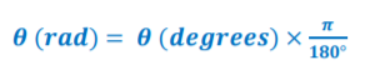Point out that if we want to convert from radians to degrees, we use this formula: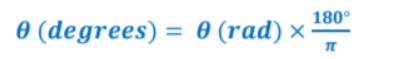Use this video to provide a worked example of how we convert radians to degrees. The video explains how we can convert 150 degrees and – 45 degrees to radians. This video is a great resource on how to convert degrees to radians.

### Length of a Sector and Area of a Sector

Point out that we know that radian is the measure of the plane angle subtended by a circular arc. In general, if an arc of length S subtends an angle θ (rad), with the radius of the circle given as r, then: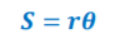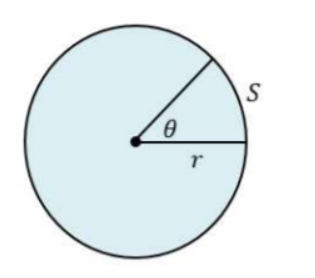When it comes to the area of a sector, explain that it’s given by this formula: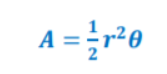Use this video in your classroom to demonstrate how to find the length of a sector using the above formula. In addition, you can play this video that contains step-by-step instructions, as well as worked examples of how to find the area of a sector.## Activities to Practice Radians and Degrees

### Pair Work

This activity will help students practice converting between degrees and minutes, converting between radians and degrees, as well as finding the area of sector or sector length. To use this activity in your class, simply print out this Assignment Worksheet (Members Only).

Pair students up and hand out the worksheets. Explain to students that the worksheets contain different exercises where they have to convert decimal degree measure to DMS form and vice versa, convert radians to degrees or degrees to radians, etc.

Students work individually to complete the exercises. Provide a few minutes for this. After they’re done, students in each pair go through the exercises together and provide feedback to each other. The activity thus also provides an opportunity for strengthening peer tutoring.

### Radians and Degrees Online Game

This is an online game that will help students practice converting from radians to degrees and from degrees to radians. Introduce this game at the end of your lesson on radians and degrees. The only thing you’ll need for this game is a number of suitable technical devices for students.

Students play the game individually, which makes it great for parents who are homeschooling their children as well. Explain to students that they need to convert between radians and degrees in the game.

Provide a few minutes for students to complete the exercises. Once they’re done, leave room for discussion and reflection on the exercises. Which formula did students use to convert radians to degrees? Which one did they use to convert degrees to radians?

## Before You Leave…

If you enjoyed these strategies and activities for teaching radians and degrees, you may want to check out our lesson that’s dedicated to this topic. So if you need guidance to structure your class and teach pre-calculus, make sure to sign up for more free resources here!

You can also sign up for our membership on MathTeacherCoach or head over to our blog – you’ll find plenty of awesome resources for kids of all ages!